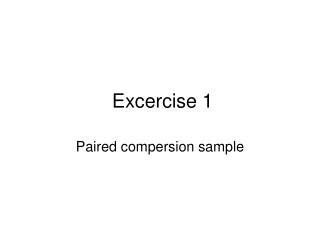DownloadDownload PresentationExcercise 1

Excercise 1

Download PresentationExcercise 1

- - - - - - - - - - - - - - - - - - - - - - - - - - - E N D - - - - - - - - - - - - - - - - - - - - - - - - - - -
Presentation Transcript

1. Excercise 1 Paired compersion sample

2. Scaling Procedure Based on the Method of Paired Comparsion • Attributes of products are not equally important for customers • We have to discover the rank of these features and thus we can focuse on the main needs of customers.

3. Exercise • Coffee: • Strong (I1) • Milky (I2) • Sweet (I3) • Hot (I4) • With Cinnamon (I5) • With whipped cream (I6) • Create pairs and Rank them randomly or use Ross-method • Compare them, underline the preferred item

4. Create Preference Matrix – this contains preferences. Both in the rows and in the cols the dimensons are presented. When someone prefer item in row to item in col there is 1, otherwise 0. • In the last col there is the sum of the value being in the row. This means how often the row item was preferred to the others.

5. Consistency test • There are 3 item: A, B, C • If A>B and B>C then A>C when the decision maker is consistent • Consistency coeeficient: • Where dmax is the maximum number of incosistent decisions • If n is odd number • If n is even number: 6 item n=6 dmax=8

6. Person 1. d=(5*5*11)/12-55/2=27,5-27,5=0 • K= 1-0/8=1 100,00% a2=55

7. Person 2 d=27,5-55/2=0 • K= 100,00% a2=55

8. Person 3 d=27,5-55/2=0 • K= 100,00% a2=55

9. Person 4 d=27,5-53/2=1 • K= 87,5% a2=53

10. Person 5 d=27,5-53/2=1 • K= 87,5% a2=53

11. Person 6 d=27,5-55/2=0 • K= 100% a2=55

12. Person 7 d=27,5-55/2=0 • K= 100% a2=55

13. Person 8 d=27,5-47/2=4 • K= 50% a2=47

14. Person 9 d=27,5-55/2=0 • K= 100% a2=55

15. Summarized preference matrix

16. Creation of weighted number • Preference fraction: Where „m” is the number of decision makers. To get the weighted number we have to transform Pa value into a normal distribution or simply calculate the percentage value of it. if m=14

17. Totalized preference matrix Pamax=0,639 Pa min=0,333 Pamax – Pamin= 0,306

18. Kendall coefficient of concordance(W) • Rj is the sum of the colums in the totalized preference matrix • is the mean of Rj-s • Δ is the squared distance • m is the number of decision maker • n is the number of items

19. W=76/630=0,12 Rjmean=15 Δ=76 Δmax=630

20. Meaning of the Kendall Coefficient • Kendall's coefficient of concordance is used traditionally in statistics for measuring agreement between k orderings. • If it is 1 there is total concordance. • If it is 0 there is no concordance at all. But it even occure in that case when there is two group of decision maker with opposite oppinion. Then cluster analyize should be used to discover the groups.

21. Thank You for Attention